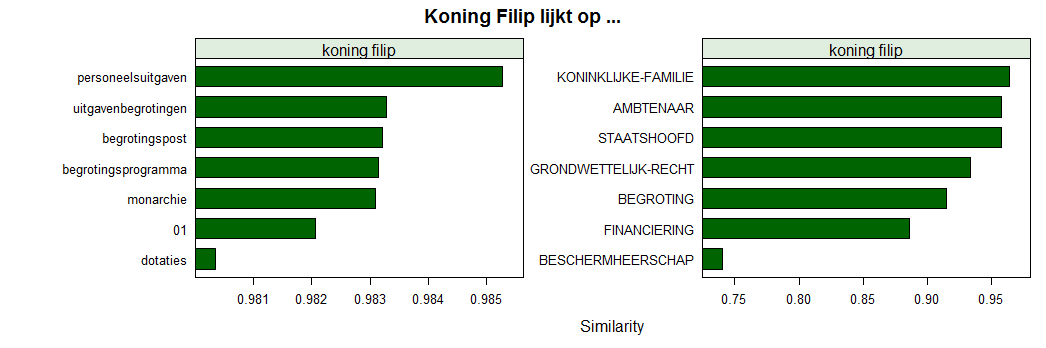[This article was first published on bnosac :: open analytical helpers - bnosac :: open analytical helpers, and kindly contributed to R-bloggers]. (You can report issue about the content on this page here)
Want to share your content on R-bloggers? click here if you have a blog, or here if you don't.

Last call for the course on Text Mining with R, held next week in Leuven, Belgium on April 1-2. Viewing the course description as well as subscription can be done at https://lstat.kuleuven.be/training/coursedescriptions/text-mining-with-r

Some things you’ll learn … is that King Filip of Belgium is similar to public expenses if we just look at open data from questions and answers in Belgian parliament (retrieved from here http://data.dekamer.be). Proof is below. See you next week.```library(ruimtehol)
library(data.table)
library(lattice)
library(latticeExtra)
data("dekamer", package = "ruimtehol")
dekamer\$x <- strsplit(dekamer\$question, "\\W")
dekamer\$x <- lapply(dekamer\$x, FUN = function(x) setdiff(x, ""))
dekamer\$x <- sapply(dekamer\$x, FUN = function(x) paste(x, collapse = " "))
dekamer\$x <- tolower(dekamer\$x)
dekamer\$y <- strsplit(dekamer\$question_theme, split = ",")
dekamer\$y <- lapply(dekamer\$y, FUN=function(x) gsub(" ", "-", x))
set.seed(321)
model <- embed_tagspace(x = dekamer\$x, y = dekamer\$y,
early_stopping = 0.8, validationPatience = 10,
dim = 50,
lr = 0.01, epoch = 40, loss = "softmax", adagrad = TRUE,
similarity = "cosine", negSearchLimit = 50,
ngrams = 2, minCount = 2)embedding_words  <- as.matrix(model, type = "words")
embedding_labels <- as.matrix(model, type = "labels", prefix = FALSE)
embedding_person <- starspace_embedding(model, tolower(c("Theo Francken")))
embedding_person <- starspace_embedding(model, tolower(c("Koning Filip")))
similarities <- embedding_similarity(embedding_person, embedding_words, top = 9)
similarities <- subset(similarities, !term2 %in% c("koning", "filip"))
similarities\$term <- factor(similarities\$term2, levels = rev(similarities\$term2))
plt1 <- barchart(term ~ similarity | term1, data = similarities,
scales = list(x = list(relation = "free"), y = list(relation = "free")),
col = "darkgreen", xlab = "Similarity", main = "Koning Filip lijkt op ...")similarities <- embedding_similarity(embedding_person, embedding_labels, top = 7)
similarities\$term <- factor(similarities\$term2, levels = rev(similarities\$term2))
plt2 <- barchart(term ~ similarity | term1, data = similarities,
scales = list(x = list(relation = "free"), y = list(relation = "free")),
col = "darkgreen", xlab = "Similarity", main = "Koning Filip lijkt op ...")
c(plt1, plt2)```